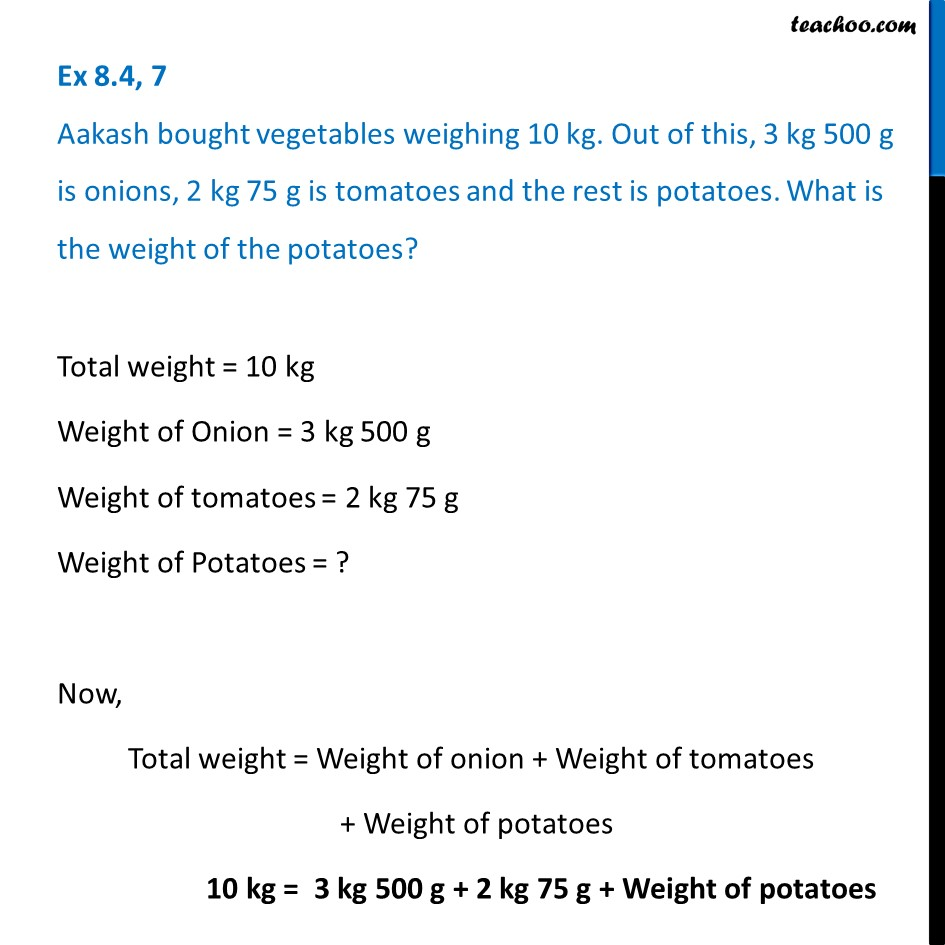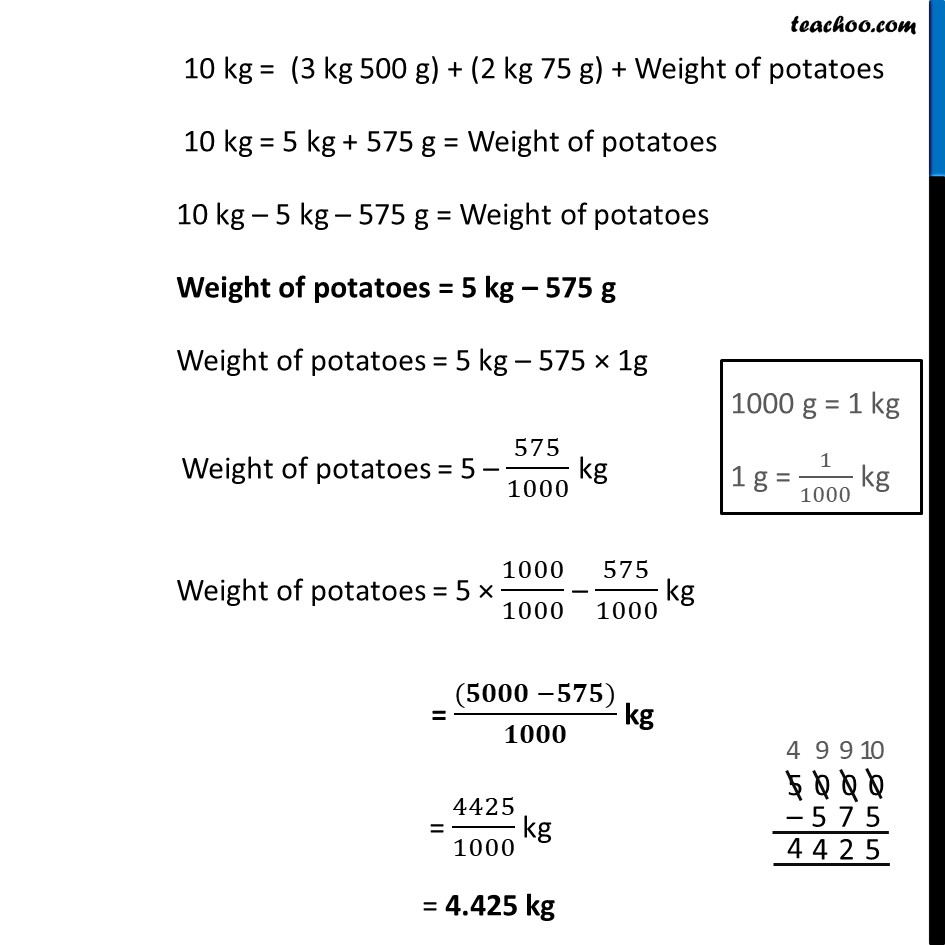Ex 8.4

Chapter 8 Class 6 Decimals
Serial order wiseLearn in your speed, with individual attention - Teachoo Maths 1-on-1 Class

### Transcript

Ex 8.4, 7 Aakash bought vegetables weighing 10 kg. Out of this, 3 kg 500 g is onions, 2 kg 75 g is tomatoes and the rest is potatoes. What is the weight of the potatoes?Total weight = 10 kg Weight of Onion = 3 kg 500 g Weight of tomatoes = 2 kg 75 g Weight of Potatoes = ? Now, Total weight = Weight of onion + Weight of tomatoes + Weight of potatoes 10 kg = 3 kg 500 g + 2 kg 75 g + Weight of potatoes 10 kg = (3 kg 500 g) + (2 kg 75 g) + Weight of potatoes 10 kg = 5 kg + 575 g = Weight of potatoes 10 kg – 5 kg – 575 g = Weight of potatoes Weight of potatoes = 5 kg – 575 g Weight of potatoes = 5 kg – 575 × 1g Weight of potatoes = 5 – 575/1000 kg Weight of potatoes = 5 × 1000/1000 – 575/1000 kg = ((𝟓𝟎𝟎𝟎 −𝟓𝟕𝟓))/𝟏𝟎𝟎𝟎 kg = 4425/1000 kg = 4.425 kg